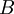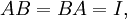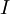Inverse matrix

In linear algebra, an square matrix$A$ is called invertible or non-singular if there exists a matrix$B$ such that$AB = BA = I, \$
where$I$ is the identity matrix.